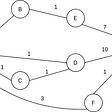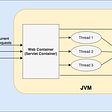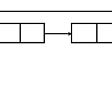# DSA #1: Big O notation Time/Space complexity

## Introduction

Big O Notation is a way to measure an algorithm’s efficiency. It measures the time it takes to run your function as the input grows. Or in other words, how well does the function scale.

There are two parts to measuring efficiency — time complexity and space complexity. Time complexity is a measure of how long the function takes to run in terms of its computational steps. Space complexity has to do with the amount of memory used by the function. In this post, I will share with you the most common runtimes you can see as a software developer.

Big O notation allows you to find the time and space complexity for the worst case.

## Most common runtimes

• O (1) : constant complexity
• O (N): Linear complexity
• O (log N): Logarithm complexity

So Let me explain to you each runtime, his graph, and show an example.

## Constant complexity O(1)

The constant complexity means no matter the input size, the runtime will be always the same, O(20) = 1, O(N) = 1.

Graph:

`arr = [0,4,5,7,9,10]function display(arr){  console.log(arr);}`

Explanation: We can see above that the array size is 6, but our function will always take the same time because it accesses directly the item that is at the second position.

## Linear complexity O(n)

The Time and space that are being taken will grow linearly according to the input size like O (1) = 1, O (n) = n.

Graph

Example:

`arr = [0,4,5,7,9,10]function browseArray(arr){    for(let i=0; i< arr.length; i++ )       // perform some operation  }}`

Explanation: In the example above, the array size is 6 we can deduce that the loop in browseArray function will make 6 tours and if the array size increase the number of loops will increase linearly.

It represents an algorithm whose performance is directly proportional to the squared size of the input data set. The time complexity will occur whenever we nest over multiple iterations. So if for example, the input grows from 3 to 4, the growth rate would change from ³² to ⁴², so 9 to 16.

Graph

Example:

`arr = [0,4,5,7,9,10]function browseArray(arr){    for(let i=0; i< arr.length; i++ )    for(let j =0; j < arr.length; j++ ){       // perform some operation    }  }}`

Explanation: The array size is 6 and for each iteration of the first loop we have an inner loop that goes to all the items again.

## Logarithm complexity O(log(N))

When the runtime of your algorithm is a logarithm complexity you can increase the number of input exponentially but the time will grow linearly O(0) = 1, O(100) = 2.

Graph

Example:

`function browseArray(arr){    for(let i=0; i< arr.length; i = i *2 )   // perform some operation  }}`

## Common runtimes with their graph

According to the graph above, we can deduce the best and the worst runtime.

In this article, we went over four complexities: linear, constant, quadratic, and logarithmic. While there are many more these are some of the most common and useful complexities to be familiar with. I hope that you learn something new by reading this article.

The Nothing 🔺

--

--

--

## More from Marius NIEMET

I am just a developer slapping the keyboard daily until magic happens. https://www.mariusniemet.me/

Love podcasts or audiobooks? Learn on the go with our new app.

## #206: Let’s Create an Isekai Anime Together With “Anime Loot”!## Golang : Dijkstra Algorithm## How To Make a Piano App in Flutter## How to Convert Excel (XLSX) Files to JSON Object Arrays using Java## Hacking Series Part 18## Enterprise Cloud Adoption: 3 Insights for Driving Change## Deploy Your Portfolio Website with Azure Static Web Apps and Azure DevOps## Marius NIEMET

I am just a developer slapping the keyboard daily until magic happens. https://www.mariusniemet.me/

## Servlet Life Cycle — Java Programing## Trees In Java Explained In Detail## Learn Queue ausing Java